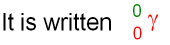gcsescience.com                                       9                                       gcsescience.com

Gamma rays.

What is a Gamma Ray?

A gamma ray (also written as γ-ray) is an electromagnetic wave.
It is at the small wavelength end of the electromagnetic spectrum.
A gamma ray has no mass and no charge.Emitting a gamma ray makes no difference to the mass number
or the atomic number but will make the nucleus more stable.

What are the Properties of a Gamma Ray
?

1. A gamma ray has no charge (see above).

2. A gamma ray has no mass. Of the three types of radioactivity
a gamma ray is the least likely to collide with other atoms
which means that

(i) gamma rays have the most penetrating ability and are not easily
absorbed. Many centimetres of lead or a few metres of concrete
will absorb most gamma rays but some will still get through.

(ii) gamma rays are the least ionising radiation.

Where do
Gamma Rays come from?

Gamma rays are likely to be emitted at the same time as
alpha particles or beta particles but
some isotopes only emit gamma rays (see some examples).

How can the Direction of a
Gamma Ray be Changed?

Unlike an alpha particle or a beta particle, a gamma ray is not
deflected by a magnetic or electric field because it has no charge.

gcsescience.com     Physics Quiz     Index     Radioactivity Quiz     gcsescience.com